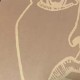# 一只菜鸡关于《Project NANO》的解谜记录

This article was published over 3年 ago. The information mentioned may be changed or developed.

この記事は 3年前に書かれたものです。記載された情報は変化した可能性があります。

## 过程

### 谜题入口（Entry）

ZeroMe Logs | Mar 26, 2020.

……RGB啊！
[时序碎片: 51 51]
    <div class="not-here">
<p>这啥？？？？</p>
<div>
<div class="color-block" style="background-color: rgb(65, 87, 69)"></div>
<div class="color-block" style="background-color: rgb(83, 79, 77)"></div>
<div class="color-block" style="background-color: rgb(69, 46, 32)"></div>
<div class="color-block" style="background-color: rgb(110, 101, 120)"></div>
<div class="color-block" style="background-color: rgb(116, 32, 58)"></div>
<div class="color-block" style="background-color: rgb(32, 47, 116)"></div>
<div class="color-block" style="background-color: rgb(119, 111, 102)"></div>
<div class="color-block" style="background-color: rgb(111, 111, 116)"></div>
</div>
<p class="what-is-this">3983153e.log</p>
</div>

AWESOME. next : /twofoot

/twofoot 就是下一步的链接了。

### Two foot

<title>Can't you see what's wrong?</title>
<!-- ... -->
<div class="not-here">
<p class="what-is-this">No hint!</p>
<p>本关的题目就在画面上</p>
<p>什么？你没看到吗？或许… 你需要去配眼镜了（讨打）</p>
<p class="what-is-this">...</p>
</div>

“Can’t you see what’s wrong?”

two foot feet

### Two feet

<div id="not-here">
<p class="what-is-this">答案用下划线代替空格，全小写</p>
<p>********，你有听说过吗？</p>
<p>它里面有首歌挺好听的，但只有短短的几秒。</p>
<p>你能帮我找到原曲吗？谢谢啦。</p>
<p>给，这是一个视频，供参考。</p>
<p class="what-is-this" onclick="ido.not.know()">视频呢？</p>
</div>
<div id="-qu" class="why-can-not-see"><pre><code>
=EA=93=9Du=E7=BD=AE=E8=BF=99h=CE=B1=D0=B8g=E8=AF=9D=C2=A2=E2=87=92=F0=9D=9F=99=F0=9D=9F=A8=D1=85=F0=9D=9F=BA=F0=9D=9F=A3=F0=9D=9F=A3=F0=9D=9F=A8=F0=9D=9F=BDrY=E2=87=90=C2=A2=E8=BD=AC=E7=A7=BB=E4=BE=84b=C3=AC=C5=82=C3=AEB=C3=AFLi..d=D0=B0k=CE=B1=C3=AC=C2=A2d=D0=BEge=E2=87=90.=E2=87=90=E5=A5=BD=E6=A9=A1b=E1=88=80=E9=92=9B=E5=AF=B9j=C3=AC=D0=B8g=EF=BC=9F
</code></pre></div>
<script>ido=[];ido.not=[];ido.not.know=function(){document.getElementById('-qu').className="";document.getElementById('not-here').className="why-can-not-see"}</script>


=EA=93=9Du=E7=BD=AE=E8=BF=99h=CE=B1=D0=B8g=E8=AF=9D=C2=A2=E2=87=92=F0=9D=9F=99=F0=9D=9F=A8=D1=85=F0=9D=9F=BA=F0=9D=9F=A3=F0=9D=9F=A3=F0=9D=9F=A8=F0=9D=9F=BDrY=E2=87=90=C2=A2=E8=BD=AC=E7=A7=BB=E4=BE=84b=C3=AC=C5=82=C3=AEB=C3=AFLi..d=D0=B0k=CE=B1=C3=AC=C2=A2d=D0=BEge=E2=87=90.=E2=87=90=E5=A5=BD=E6=A9=A1b=E1=88=80=E9=92=9B=E5=AF=B9j=C3=AC=D0=B8g=EF=BC=9F

ꓝu置这hαиg话¢⇒𝟙𝟨х𝟺𝟣𝟣𝟨𝟽rY⇐¢转移侄bìłîBïLi..dаkαì¢dоge⇐.⇐好橡bሀ钛对jìиg？

### Tears From Heaven

<main class="content">
<p class="domain">🤔<span class="hidden">.clang.pp.ua</span></p>
<p class="hidden">bd12b4bc.log</p>
</main>

ZeroMe Logs | Mar 31, 2020.
abc 22:31:12

Piggy 22:32:10

abc 22:33:34

Piggy 22:35:58

abc 22:36:41

[解锁新的文章碎片： aHR0cHM6Ly9oZWxwLmFsaXl1bi5jb20va25vd2xlZGdlX2RldGFpbC8yOTcyNS5odG1s]
[时序碎片: 30 31]

「文章碎片」是一个 Base64 编码的网址，文章主要介绍的 DNS 解析设置教程。

dig xn--wp9h.clang.pp.ua txt
; <<>> DiG 9.10.6 <<>> xn--wp9h.clang.pp.ua txt
;; global options: +cmd
;; ->>HEADER<<- opcode: QUERY, status: NOERROR, id: 64045
;; flags: qr rd ra; QUERY: 1, ANSWER: 1, AUTHORITY: 0, ADDITIONAL: 1
;; OPT PSEUDOSECTION:
; EDNS: version: 0, flags:; udp: 512
;; QUESTION SECTION:
;xn--wp9h.clang.pp.ua.		IN	TXT
xn--wp9h.clang.pp.ua.	299	IN	TXT	"https://clang.pp.ua/Simp1E_Dec"
;; Query time: 284 msec
;; SERVER: 8.8.8.8#53(8.8.8.8)
;; WHEN: Sun Apr 12 12:52:36 UTC 2020
;; MSG SIZE  rcvd: 92

### Simple dec

<main class="content">
<p class="question">?</p>
<br />
<br />
<button class="submit">Submit!</button>
<br />
<p class="hidden">87bbdc78.log</p>
</main>
<input type="text" class="fake-input" />
<script>
const inputBox = document.querySelector(".input");
document.querySelector(".fake-input").focus();
});
const path = magic("\xef\xbb\xe4\xb1\xd9\xc2\xd3\xfc\xf7\xf9", inputBox.value);
if (!/^[0-9A-Za-z_\-]+$/.test(path)) { alert("Wrong Answer!") } else { // However, there's still a chance that you entered a wrong answer; location.pathname = "/" + path; } }); </script> 那段混淆 JS 的内容放在这里了。放在博客里面直接渲染有点炸。 「日志」内容： ZeroMe Logs | Apr 1, 2020. abc 00:21:22 小猪啊，现在这里又有一个文本框，出题人告诉我输入密钥就能通关。 Piggy 00:22:10 是啊，我只知道密钥是 Talion ，但是好像输不进去的样子。 abc 00:23:58 对，有什么东西阻挡住了，得想办法移走他。 Piggy 00:24:42 …… [时序碎片: 31 35] 基本上看代码逻辑的话，里面有一段： if (!/^[0-9A-Za-z_\-]+$/.test(path)) {
}

，发表于

## 评论

### 《“一只菜鸡关于《Project NANO》的解谜记录”》 有 2 条评论

1.大学学的信息安全，就像是其他专业有acm，每年学长都会给我们准备ctf的题。
回想起来当时和同学通宵做题抢分，真是有意思。
一转眼都毕业三年了。

2. […] 之前写过了一篇选手视角的题解，亦可以作为参考：一只菜鸡关于《Project NANO》的解谜记录 […]